# q4week 3.pptx

30. May 2023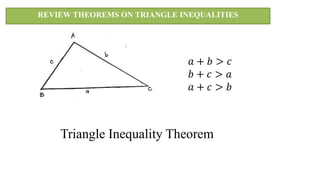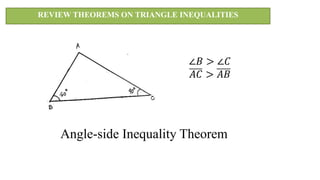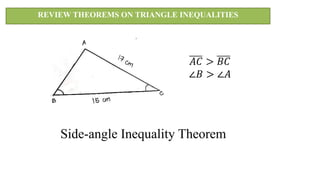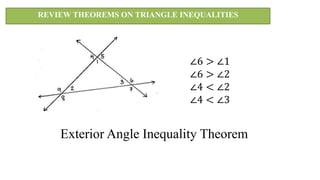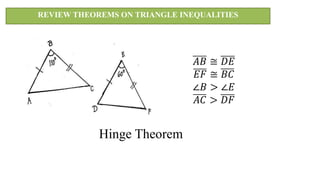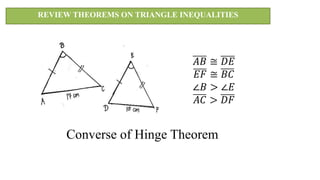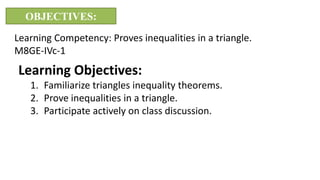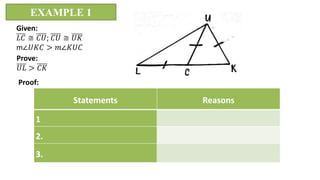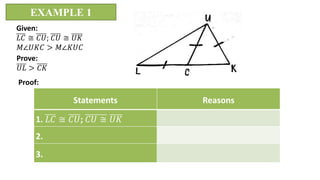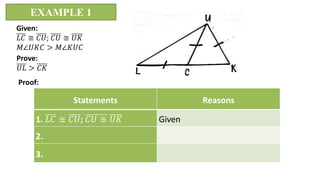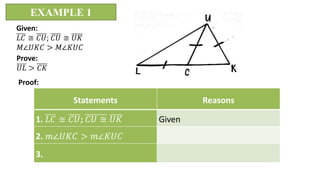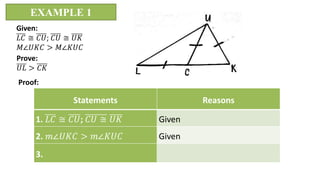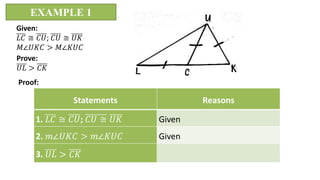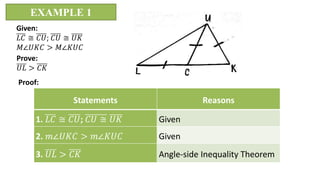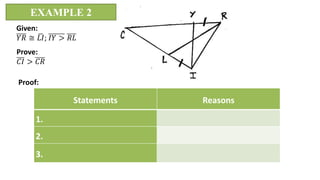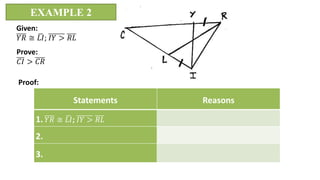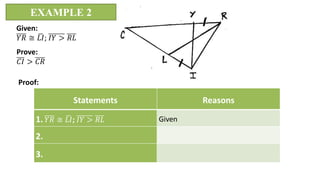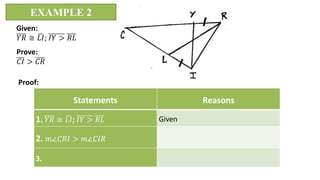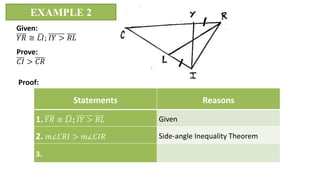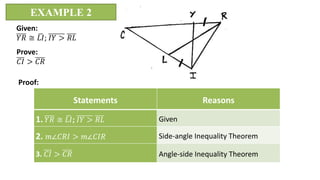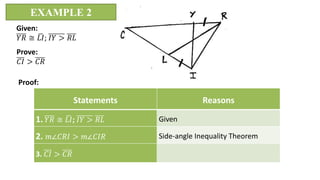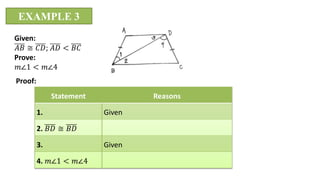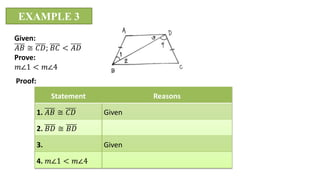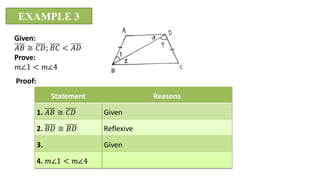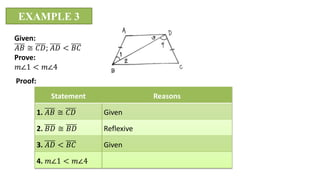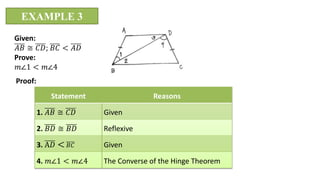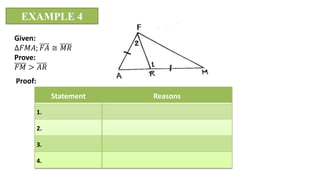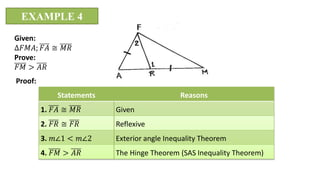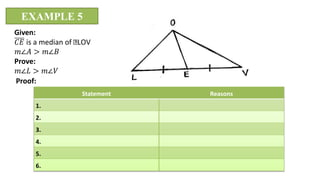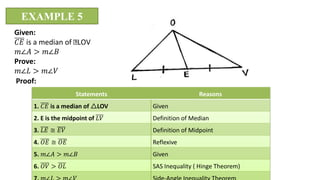1 von 30

### q4week 3.pptx

• 1. REVIEW THEOREMS ON TRIANGLE INEQUALITIES 𝑎 + 𝑏 > 𝑐 𝑏 + 𝑐 > 𝑎 𝑎 + 𝑐 > 𝑏 Triangle Inequality Theorem
• 2. REVIEW THEOREMS ON TRIANGLE INEQUALITIES ∠𝐵 > ∠𝐶 𝐴𝐶 > 𝐴𝐵 Angle-side Inequality Theorem
• 3. REVIEW THEOREMS ON TRIANGLE INEQUALITIES 𝐴𝐶 > 𝐵𝐶 ∠𝐵 > ∠𝐴 Side-angle Inequality Theorem
• 4. REVIEW THEOREMS ON TRIANGLE INEQUALITIES ∠6 > ∠1 ∠6 > ∠2 ∠4 < ∠2 ∠4 < ∠3 Exterior Angle Inequality Theorem
• 5. REVIEW THEOREMS ON TRIANGLE INEQUALITIES 𝐴𝐵 ≅ 𝐷𝐸 𝐸𝐹 ≅ 𝐵𝐶 ∠𝐵 > ∠𝐸 𝐴𝐶 > 𝐷𝐹 Hinge Theorem
• 6. REVIEW THEOREMS ON TRIANGLE INEQUALITIES 𝐴𝐵 ≅ 𝐷𝐸 𝐸𝐹 ≅ 𝐵𝐶 ∠𝐵 > ∠𝐸 𝐴𝐶 > 𝐷𝐹 Converse of Hinge Theorem
• 7. OBJECTIVES: Learning Competency: Proves inequalities in a triangle. M8GE-IVc-1 Learning Objectives: 1. Familiarize triangles inequality theorems. 2. Prove inequalities in a triangle. 3. Participate actively on class discussion.
• 8. EXAMPLE 1 Given: 𝐿𝐶 ≅ 𝐶𝑈; 𝐶𝑈 ≅ 𝑈𝐾 m∠𝑈𝐾𝐶 > 𝑚∠𝐾𝑈𝐶 Prove: 𝑈𝐿 > 𝐶𝐾 Proof: Statements Reasons 1 2. 3.
• 9. EXAMPLE 1 Given: 𝐿𝐶 ≅ 𝐶𝑈; 𝐶𝑈 ≅ 𝑈𝐾 𝑀∠𝑈𝐾𝐶 > 𝑀∠𝐾𝑈𝐶 Prove: 𝑈𝐿 > 𝐶𝐾 Proof: Statements Reasons 1. 𝐿𝐶 ≅ 𝐶𝑈; 𝐶𝑈 ≅ 𝑈𝐾 2. 3.
• 10. EXAMPLE 1 Given: 𝐿𝐶 ≅ 𝐶𝑈; 𝐶𝑈 ≅ 𝑈𝐾 𝑀∠𝑈𝐾𝐶 > 𝑀∠𝐾𝑈𝐶 Prove: 𝑈𝐿 > 𝐶𝐾 Proof: Statements Reasons 1. 𝐿𝐶 ≅ 𝐶𝑈; 𝐶𝑈 ≅ 𝑈𝐾 Given 2. 3.
• 11. EXAMPLE 1 Given: 𝐿𝐶 ≅ 𝐶𝑈; 𝐶𝑈 ≅ 𝑈𝐾 𝑀∠𝑈𝐾𝐶 > 𝑀∠𝐾𝑈𝐶 Prove: 𝑈𝐿 > 𝐶𝐾 Proof: Statements Reasons 1. 𝐿𝐶 ≅ 𝐶𝑈; 𝐶𝑈 ≅ 𝑈𝐾 Given 2. 𝑚∠𝑈𝐾𝐶 > 𝑚∠𝐾𝑈𝐶 3.
• 12. EXAMPLE 1 Given: 𝐿𝐶 ≅ 𝐶𝑈; 𝐶𝑈 ≅ 𝑈𝐾 𝑀∠𝑈𝐾𝐶 > 𝑀∠𝐾𝑈𝐶 Prove: 𝑈𝐿 > 𝐶𝐾 Proof: Statements Reasons 1. 𝐿𝐶 ≅ 𝐶𝑈; 𝐶𝑈 ≅ 𝑈𝐾 Given 2. 𝑚∠𝑈𝐾𝐶 > 𝑚∠𝐾𝑈𝐶 Given 3.
• 13. EXAMPLE 1 Given: 𝐿𝐶 ≅ 𝐶𝑈; 𝐶𝑈 ≅ 𝑈𝐾 𝑀∠𝑈𝐾𝐶 > 𝑀∠𝐾𝑈𝐶 Prove: 𝑈𝐿 > 𝐶𝐾 Proof: Statements Reasons 1. 𝐿𝐶 ≅ 𝐶𝑈; 𝐶𝑈 ≅ 𝑈𝐾 Given 2. 𝑚∠𝑈𝐾𝐶 > 𝑚∠𝐾𝑈𝐶 Given 3. 𝑈𝐿 > 𝐶𝐾
• 14. EXAMPLE 1 Given: 𝐿𝐶 ≅ 𝐶𝑈; 𝐶𝑈 ≅ 𝑈𝐾 𝑀∠𝑈𝐾𝐶 > 𝑀∠𝐾𝑈𝐶 Prove: 𝑈𝐿 > 𝐶𝐾 Proof: Statements Reasons 1. 𝐿𝐶 ≅ 𝐶𝑈; 𝐶𝑈 ≅ 𝑈𝐾 Given 2. 𝑚∠𝑈𝐾𝐶 > 𝑚∠𝐾𝑈𝐶 Given 3. 𝑈𝐿 > 𝐶𝐾 Angle-side Inequality Theorem
• 15. EXAMPLE 2 Given: 𝑌𝑅 ≅ 𝐿𝐼; 𝐼𝑌 > 𝑅𝐿 Prove: 𝐶𝐼 > 𝐶𝑅 Proof: Statements Reasons 1. 2. 3.
• 16. EXAMPLE 2 Given: 𝑌𝑅 ≅ 𝐿𝐼; 𝐼𝑌 > 𝑅𝐿 Prove: 𝐶𝐼 > 𝐶𝑅 Proof: Statements Reasons 1. 𝑌𝑅 ≅ 𝐿𝐼; 𝐼𝑌 > 𝑅𝐿 2. 3.
• 17. EXAMPLE 2 Given: 𝑌𝑅 ≅ 𝐿𝐼; 𝐼𝑌 > 𝑅𝐿 Prove: 𝐶𝐼 > 𝐶𝑅 Proof: Statements Reasons 1. 𝑌𝑅 ≅ 𝐿𝐼; 𝐼𝑌 > 𝑅𝐿 Given 2. 3.
• 18. EXAMPLE 2 Given: 𝑌𝑅 ≅ 𝐿𝐼; 𝐼𝑌 > 𝑅𝐿 Prove: 𝐶𝐼 > 𝐶𝑅 Proof: Statements Reasons 1. 𝑌𝑅 ≅ 𝐿𝐼; 𝐼𝑌 > 𝑅𝐿 Given 2. 𝑚∠𝐶𝑅𝐼 > 𝑚∠𝐶𝐼𝑅 3.
• 19. EXAMPLE 2 Given: 𝑌𝑅 ≅ 𝐿𝐼; 𝐼𝑌 > 𝑅𝐿 Prove: 𝐶𝐼 > 𝐶𝑅 Proof: Statements Reasons 1. 𝑌𝑅 ≅ 𝐿𝐼; 𝐼𝑌 > 𝑅𝐿 Given 2. 𝑚∠𝐶𝑅𝐼 > 𝑚∠𝐶𝐼𝑅 Side-angle Inequality Theorem 3.
• 20. EXAMPLE 2 Given: 𝑌𝑅 ≅ 𝐿𝐼; 𝐼𝑌 > 𝑅𝐿 Prove: 𝐶𝐼 > 𝐶𝑅 Proof: Statements Reasons 1. 𝑌𝑅 ≅ 𝐿𝐼; 𝐼𝑌 > 𝑅𝐿 Given 2. 𝑚∠𝐶𝑅𝐼 > 𝑚∠𝐶𝐼𝑅 Side-angle Inequality Theorem 3. 𝐶𝐼 > 𝐶𝑅 Angle-side Inequality Theorem
• 21. EXAMPLE 2 Given: 𝑌𝑅 ≅ 𝐿𝐼; 𝐼𝑌 > 𝑅𝐿 Prove: 𝐶𝐼 > 𝐶𝑅 Proof: Statements Reasons 1. 𝑌𝑅 ≅ 𝐿𝐼; 𝐼𝑌 > 𝑅𝐿 Given 2. 𝑚∠𝐶𝑅𝐼 > 𝑚∠𝐶𝐼𝑅 Side-angle Inequality Theorem 3. 𝐶𝐼 > 𝐶𝑅
• 22. EXAMPLE 3 Given: 𝐴𝐵 ≅ 𝐶𝐷; 𝐴𝐷 < 𝐵𝐶 Prove: 𝑚∠1 < 𝑚∠4 Proof: Statement Reasons 1. Given 2. 𝐵𝐷 ≅ 𝐵𝐷 3. Given 4. 𝑚∠1 < 𝑚∠4
• 23. EXAMPLE 3 Given: 𝐴𝐵 ≅ 𝐶𝐷; 𝐵𝐶 < 𝐴𝐷 Prove: 𝑚∠1 < 𝑚∠4 Proof: Statement Reasons 1. 𝐴𝐵 ≅ 𝐶𝐷 Given 2. 𝐵𝐷 ≅ 𝐵𝐷 3. Given 4. 𝑚∠1 < 𝑚∠4
• 24. EXAMPLE 3 Given: 𝐴𝐵 ≅ 𝐶𝐷; 𝐵𝐶 < 𝐴𝐷 Prove: 𝑚∠1 < 𝑚∠4 Proof: Statement Reasons 1. 𝐴𝐵 ≅ 𝐶𝐷 Given 2. 𝐵𝐷 ≅ 𝐵𝐷 Reflexive 3. Given 4. 𝑚∠1 < 𝑚∠4
• 25. EXAMPLE 3 Given: 𝐴𝐵 ≅ 𝐶𝐷; 𝐴𝐷 < 𝐵𝐶 Prove: 𝑚∠1 < 𝑚∠4 Proof: Statement Reasons 1. 𝐴𝐵 ≅ 𝐶𝐷 Given 2. 𝐵𝐷 ≅ 𝐵𝐷 Reflexive 3. 𝐴𝐷 < 𝐵𝐶 Given 4. 𝑚∠1 < 𝑚∠4
• 26. EXAMPLE 3 Given: 𝐴𝐵 ≅ 𝐶𝐷; 𝐵𝐶 < 𝐴𝐷 Prove: 𝑚∠1 < 𝑚∠4 Proof: Statement Reasons 1. 𝐴𝐵 ≅ 𝐶𝐷 Given 2. 𝐵𝐷 ≅ 𝐵𝐷 Reflexive 3. A𝐷 < 𝐵𝐶 Given 4. 𝑚∠1 < 𝑚∠4 The Converse of the Hinge Theorem
• 27. EXAMPLE 4 Given: ∆𝐹𝑀𝐴; 𝐹𝐴 ≅ 𝑀𝑅 Prove: 𝐹𝑀 > 𝐴𝑅 Proof: Statement Reasons 1. 2. 3. 4.
• 28. EXAMPLE 4 Given: ∆𝐹𝑀𝐴; 𝐹𝐴 ≅ 𝑀𝑅 Prove: 𝐹𝑀 > 𝐴𝑅 Proof: Statements Reasons 1. 𝐹𝐴 ≅ 𝑀𝑅 Given 2. 𝐹𝑅 ≅ 𝐹𝑅 Reflexive 3. 𝑚∠1 < 𝑚∠2 Exterior angle Inequality Theorem 4. 𝐹𝑀 > 𝐴𝑅 The Hinge Theorem (SAS Inequality Theorem)
• 29. EXAMPLE 5 Given: 𝐶𝐸 is a median of LOV 𝑚∠𝐴 > 𝑚∠𝐵 Prove: 𝑚∠𝐿 > 𝑚∠𝑉 Proof: Statement Reasons 1. 2. 3. 4. 5. 6.
• 30. EXAMPLE 5 Given: 𝐶𝐸 is a median of LOV 𝑚∠𝐴 > 𝑚∠𝐵 Prove: 𝑚∠𝐿 > 𝑚∠𝑉 Proof: Statements Reasons 1. 𝐶𝐸 is a median of LOV Given 2. E is the midpoint of 𝐿𝑉 Definition of Median 3. 𝐿𝐸 ≅ 𝐸𝑉 Definition of Midpoint 4. 𝑂𝐸 ≅ 𝑂𝐸 Reflexive 5. 𝑚∠𝐴 > 𝑚∠𝐵 Given 6. 𝑂𝑉 > 𝑂𝐿 SAS Inequality ( Hinge Theorem)